##CS 660: Combinatorial Algorithms Intro To Binary Search Trees

[To Lecture Notes Index]
San Diego State University -- This page last updated Sept. 18, 1995## References

Introduction to Algorithms by Cormen, Leiserson, Rivest. Chapter 13

Data Structures and Algorithms 1: Sorting and Searching, Mehlhorn, Kurt, pages 174-177

## Search Trees

Access (k, t): Return the item in tree t with key k; if no item in t has key k, return null

Insert (j, t): Insert item j into t, not previously containing j

Delete (j, t): Delete item j from t

Minimum (t): Return the item in t with the smallest key

Maximum (t): Return the item in t with the largest key

Successor (k, t): Return the item in t with the smallest key larger than k

Predecessor (k, t): Return the item in t with the largest key smaller than k

Join (a, i, b): Return tree formed by combining tree a, item i, and tree b. Assumes that every item in a has key less then key(i) and every item in b has key larger than key(i)

Split (i, s): Split tree s, containing item i, into three trees: a, containing all items with key less than key(i); single tree i; and b, containing all items with key greater than key(i)

Types of Algorithms

Off-line
Totally balanced BST
Know all items in list
Minimize worst case search
Optimum BST
Know all items and probability of access
Minimize total search cost over all access

On-line
Balanced BST (Weight, Height), Skip lists
Add items as they show up
Minimize worst case search
Self-Adjusting BST
Modify tree based on access pattern

Tree Operations on BST
Access, Minimum, Maximum, Successor, Predecessor, InsertTree Operations
Delete
Case 1 Delete leaf
Delete(20)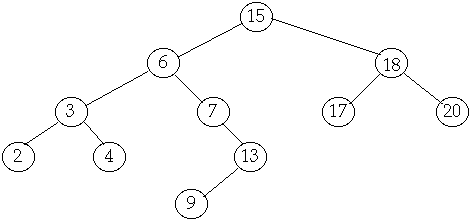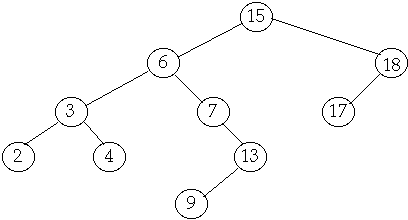Tree Operations
Delete
Case 2 Delete node with one child
Delete(7)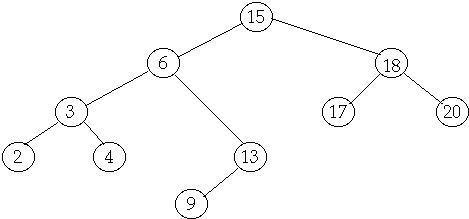Tree Operations
Delete
Case 3 Delete node with two children
Delete(6)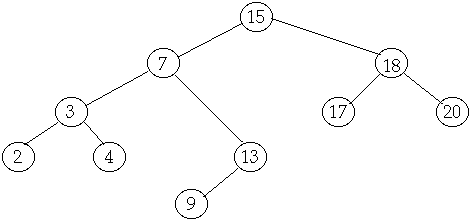### Tree Operations - Performance

Theorem
The operations Access, Minimum, Maximum, Successor, Predecessor, Insert, and delete run in O(h) on a BST of height h

Randomly Built BST

Assume that we have n distinct keys in random order

Each n! permutations of the input keys is equally likely

Insert the keys in an empty tree, using random order

Theorem
The average height of a randomly built BST on n distinct keys is O( lgn )

### General Terms

We have a list of n items: a1, a2, ..., an in BST

Probability of accessing item ak is P(ak) = Alphak

Let Betak be the probability of accessing a key that is between ak and ak+1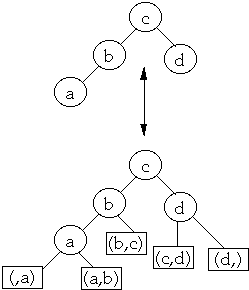Let bk be the leaf between ak and ak+1

Betak is the probability of accessing leaf bk

Ordered by keys we have , b0< a1 <b1< a2 < ... < an < bn

(Beta0 ,Alpha1 ,Beta1 , Alpha2, Beta2,... , Alphan, Betan)1 is called the access distribution

Let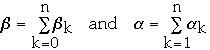Weighted path length of a tree

Let D(ak) = depth of node ak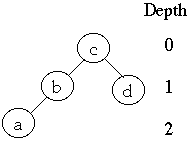Define the weighted path length of tree T as: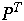is the average number of comparisons in a search of T
Entropy

Let (Gamma1 ,Gamma2,... , Gamman) be a discrete probability distribution, i.e.

Gammak >= 0 and SigmaGammak =1

H(Gamma1 ,Gamma2,... , Gamman) =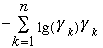is the entropy of the distribution.

Use convention that 0*lg 0 = 0

Lower bound on weighted Path Length

We have a list of n items: a1, a2, ..., an in BST

Probability of accessing item ak is P(ak) = Alphak

Let bk be the leaf between ak and ak+1

Betak is the probability of accessing leaf bk

LetH = H(Beta0 ,Alpha1 ,Beta1 , Alpha2, Beta2,... , Alphan, Betan)

P =Theorem 5 For any BST we have:
a)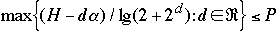b)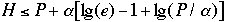Self Organizing Linear List and BST

Is there the equivalent of Move-to-Front, Transpose and Optimal Static Ordering in BST?